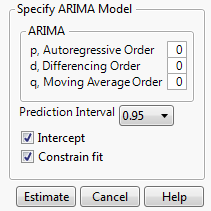Publication date: 05/24/2021

## ARIMA and Seasonal ARIMA Models

ARIMA

Shows the ARIMA Specification window, which enables you to specify the ARIMA model that you want to fit. An ARIMA model predicts future values of a time series by a linear combination of its past values and a series of errors (also known as random shocks or innovations). The ARIMA model performs a maximum likelihood fit of the specified ARIMA model to the time series. See ARIMA Model.

Note: An ARIMA model is commonly denoted ARIMA(p,d,q). If any of p, d, or q are zero, the corresponding letters are often dropped. For example, if p and d are zero, then the model would simply be a moving average model, denoted as MA(q).

Figure 18.6 ARIMA Specification Windowp, Autoregressive Order

The order p of the polynomial ϕ(B) operator.

d, Differencing Order

The order d of the differencing operator.

q, Moving Average Order

The order q of the differencing operator θ(B).

Prediction Interval

Enables you to set the prediction level between 0 and 1 for the forecast prediction intervals.

Intercept

Determines whether the intercept term μ is a part of the model.

Constrain fit

If checked, the fitting procedure constrains the autoregressive parameters to always remain within the stable region and the moving average parameters within the invertible region.

Tip: Deselect the Constrain fit option if the fitter is having difficulty finding the true optimum or if you want to speed up the fit. You can use the Model Summary table to see whether the resulting fitted model is stable and invertible.

Once you specify the model and click Estimate, a Model Report is added to the report window. See Reports.

Seasonal ARIMA

Shows the Seasonal ARIMA Specification window, which enables you to specify the Seasonal ARIMA model that you want to fit. This window has the same elements as the ARIMA specification window, but it also contains the seasonal element specifications. The additional Observations per Period option enables you to specify the number of observations per period, denoted as s. For more information about the Seasonal ARIMA model, see Seasonal ARIMA Model.

Note: Seasonal ARIMA models are denoted as Seasonal ARIMA(p,d,q)(P,D,Q)s.

Once you specify the model and click Estimate, a Model Report is added to the report window. See Reports.